Semiconductor Diode NAT Level - 1

# Semiconductor Diode NAT Level - 1

Test Description

## 10 Questions MCQ Test Topic wise Tests for IIT JAM Physics | Semiconductor Diode NAT Level - 1

Semiconductor Diode NAT Level - 1 for IIT JAM 2023 is part of Topic wise Tests for IIT JAM Physics preparation. The Semiconductor Diode NAT Level - 1 questions and answers have been prepared according to the IIT JAM exam syllabus.The Semiconductor Diode NAT Level - 1 MCQs are made for IIT JAM 2023 Exam. Find important definitions, questions, notes, meanings, examples, exercises, MCQs and online tests for Semiconductor Diode NAT Level - 1 below.
Solutions of Semiconductor Diode NAT Level - 1 questions in English are available as part of our Topic wise Tests for IIT JAM Physics for IIT JAM & Semiconductor Diode NAT Level - 1 solutions in Hindi for Topic wise Tests for IIT JAM Physics course. Download more important topics, notes, lectures and mock test series for IIT JAM Exam by signing up for free. Attempt Semiconductor Diode NAT Level - 1 | 10 questions in 30 minutes | Mock test for IIT JAM preparation | Free important questions MCQ to study Topic wise Tests for IIT JAM Physics for IIT JAM Exam | Download free PDF with solutions
 1 Crore+ students have signed up on EduRev. Have you?
*Answer can only contain numeric values
Semiconductor Diode NAT Level - 1 - Question 1

### A 60 V peak full wave rectified voltage is applied to a capacitor input filter. If f = 120Hz, RL=10Ω and C=10μF, the ripple voltage (V) is :

Detailed Solution for Semiconductor Diode NAT Level - 1 - Question 1

Peak to peak ripple voltage for capacitor input filter,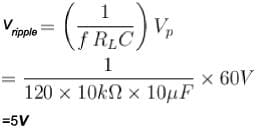*Answer can only contain numeric values
Semiconductor Diode NAT Level - 1 - Question 2

### If a zener diode (Vz = 5V and lz = 10 mA) is connected in series with a resistance and 20V is applied across the combination, then the maximum resistance one can use without spoiling zener action (in kΩ) is :

Detailed Solution for Semiconductor Diode NAT Level - 1 - Question 2

The principal use of a zener diode is in a voltage regulator. In a voltage regulator, the voltage across the load remains constant over a range of current.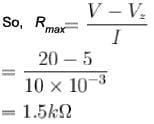*Answer can only contain numeric values
Semiconductor Diode NAT Level - 1 - Question 3

### If the peak output voltage of a full-wave rectifier is 10V, its dc voltage (V) is :

Detailed Solution for Semiconductor Diode NAT Level - 1 - Question 3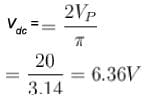*Answer can only contain numeric values
Semiconductor Diode NAT Level - 1 - Question 4

For the rectifier circuit shown in figure, the sinusoidal voltage (V1 or V2) at the output transformer has a maximum value of 10 V. The load resistance RL is 1kΩ. What is the value of lav as a multiple of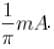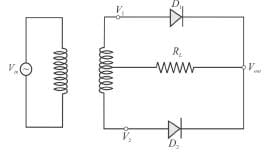Detailed Solution for Semiconductor Diode NAT Level - 1 - Question 4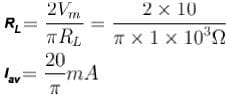*Answer can only contain numeric values
Semiconductor Diode NAT Level - 1 - Question 5

What is the diode current (A) in the circuit: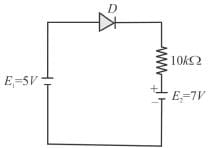Detailed Solution for Semiconductor Diode NAT Level - 1 - Question 5

Diode will be in conducting state, when forward biased voltage is applied to it and in non-conducting state, when reverse biased voltage is applied to it. Now, the two batteries may be replaced by a single battery of 2Vwhich will make the diode reverse biased and diode goes to non-conducting state and hence current is zero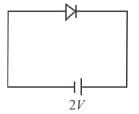*Answer can only contain numeric values
Semiconductor Diode NAT Level - 1 - Question 6

A zener diode has a breakdown voltage of 9.1 V with maximum power dissipation of 364mW. What the maximum current A that the diode can withstand?

Detailed Solution for Semiconductor Diode NAT Level - 1 - Question 6

Maximum current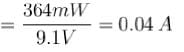*Answer can only contain numeric values
Semiconductor Diode NAT Level - 1 - Question 7

For a zener diode, Vz = 20 V and power rating of 0.2W. If the source battery provides a potential difference of 50 V. The minimum value of Rs in Ω that prevents zener diode from being destroyed is.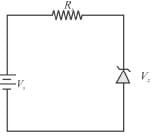Detailed Solution for Semiconductor Diode NAT Level - 1 - Question 7

Maximum zener current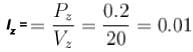Minimum value of Rs=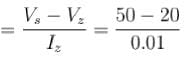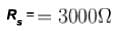*Answer can only contain numeric values
Semiconductor Diode NAT Level - 1 - Question 8

A silicon diode is in series with a 1.0kΩ resistor and a 5V battery. If the node is connected to the positive battery terminal, the cathode voltage with respect to the negative battery terminal is (in Volts)

Detailed Solution for Semiconductor Diode NAT Level - 1 - Question 8

The cathode voltage with respect to the negative battery terminal is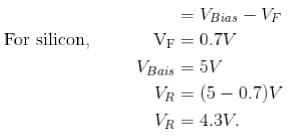*Answer can only contain numeric values
Semiconductor Diode NAT Level - 1 - Question 9

If RL = 1kΩ, find the maximum zener current (in mA).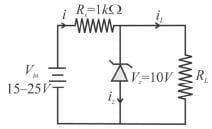Detailed Solution for Semiconductor Diode NAT Level - 1 - Question 9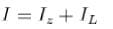Voltage developed across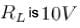always.
For maximum current l (and hence also zener current lz),Vin = 25V.
Applying KVL across loop 1, we have
(25 -10 ) = 10 x 103 x l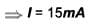Hence, maximum zener current.
lz = (15 - 10)
= 5mA

*Answer can only contain numeric values
Semiconductor Diode NAT Level - 1 - Question 10

Assuming that the two diode D1 and D2 used in the electric circuit shown in the figure are ideal, find out the value of the current flowing through 1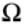resistor.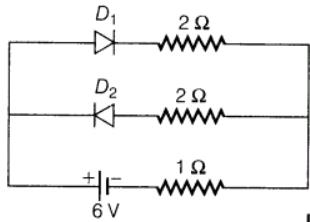Detailed Solution for Semiconductor Diode NAT Level - 1 - Question 10

According to the question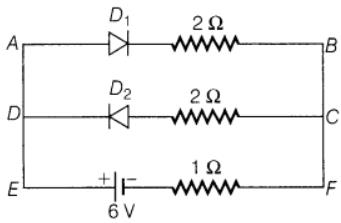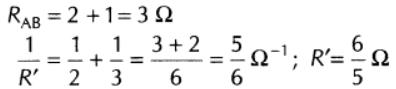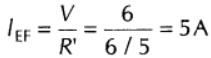## Topic wise Tests for IIT JAM Physics

217 tests
Information about Semiconductor Diode NAT Level - 1 Page
In this test you can find the Exam questions for Semiconductor Diode NAT Level - 1 solved & explained in the simplest way possible. Besides giving Questions and answers for Semiconductor Diode NAT Level - 1, EduRev gives you an ample number of Online tests for practice

## Topic wise Tests for IIT JAM Physics

217 tests(Scan QR code)